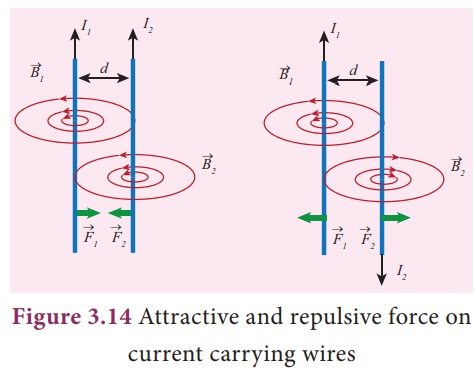Home | | Science 9th std | Force on parallel current carrying conductors

# Force on parallel current carrying conductors

Using Flemingâ€™s le hand rule we can nd that the direction of the force on each wire would be towards each other when the current in both of them are owing in the same direction. at is the wires would experience an attractive force.

Force on parallel current carrying conductors

We have seen that a current carrying conductor has a magnetic field around it. If we place another conductor carrying current parallel to the first one, the second conductor will experience a force due to the magnetic field of the first conductor. Similarly, the first conductor will experience a force due to the magnetic field of the second conductor. These two forces will be equal in magnitude and opposite in direction. This is shown in Fig. 3.14.Using Flemingâ€™s le hand rule we can nd that the direction of the force on each wire would be towards each other when the current in both of them are owing in the same direction. at is the wires would experience an attractive force. However, if the direction of the flow of current is in opposite direction, then the force on each of the wire will also be in opposite direction. ese are shown in Figure 3.14. The perpendicular view of the same is shown in Figure 3.15.## Connection between Electricity and magnetism:

Before 18th century people thought that magnetism and electricity were separate subjects of study. A er Oersted experiment the electricity and magnetism were united and became a single subject called â€śElectromagnetismâ€ť.

When there is current, the magnetic field is produced and the current carrying conductor behaves like a magnet. You may now wonder how was it possible for a lodestone to behave like a magnet when there was no current passing through it. In the twentieth century only we understood that the magnetic property arises due to the motion of electrons in the lodestone. In the circuit the electrons flow from negative of the battery to positive of the battery and constitutes current. As a result it produces magnetic field. In natural magnets and artificial magnets we buy in shops, the electrons move around the nucleus constitutes current which leads to magnetic property. Here, every orbiting electron in its orbit is like a current carrying loop. Even though in all materials the electron orbits around the nucleus, only for certain special type of material called magnetic material the motion of electrons around the nucleus gets added up and as a result we have permanent magnetic field.

Study Material, Lecturing Notes, Assignment, Reference, Wiki description explanation, brief detail
9th Science : Magnetism and Electromagnetism : Force on parallel current carrying conductors |